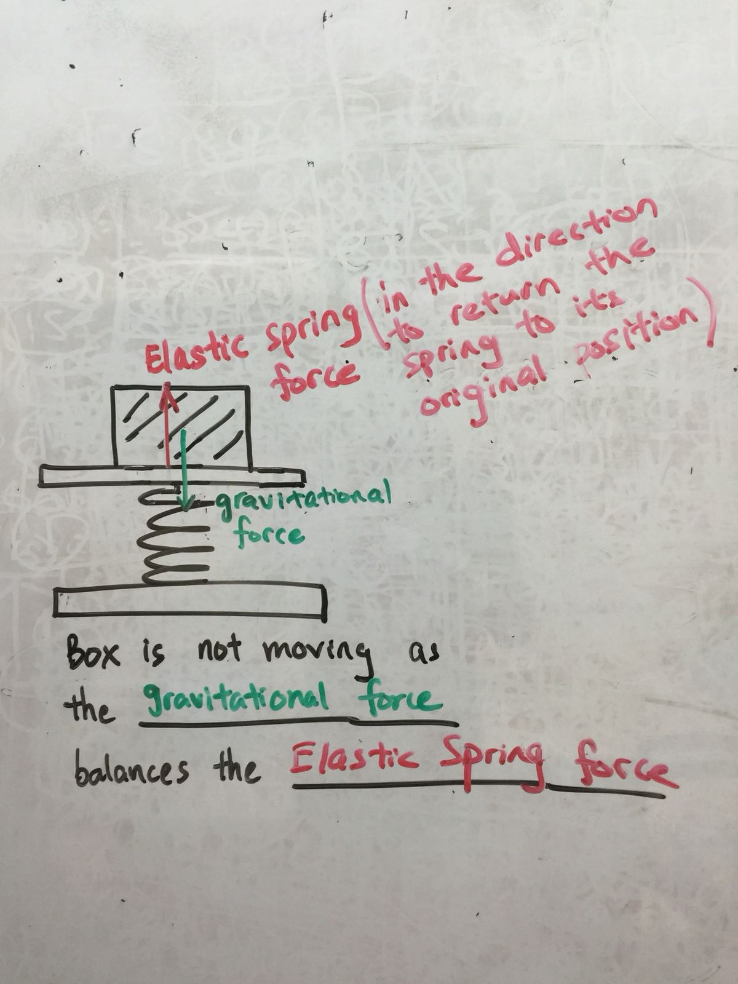# Question

Hi,

Couldn’t load the picture. The picture shows a spring with a weight placed on top of the spring.  The spring is compressed.

Draw and name the two forces acting on the spring.

Thanks in advance.

2 Answers

# Answer

Hi GBU!

In this case, because the question is specifically asking about the forces acting ON the spring (as opposed to the forces from the spring & weight), the answer you’ve gotten is likely to be correct.

For one, we know that gravity is force that helps us keep things on the ground. In this case, the first down arrow is for gravity, which keeps the spring on the ground.

The second down arrow of “Gravitational Potential Energy” can also be termed as “Weight”, because our weight is actually just a measurement of the GPE that is acting on us! That is why our weight on the moon would be lesser than our weight on Earth, because there is less gravity and hence less GPE acting on us when we are on the moon.

I hope this helps answer your question, and I apologise if the diagram confused you as it was meant to depict the forces acting in both directions (misunderstood the question, sorry!)

-Michelle (www.facebook.com/dingletutors)

0 Replies 0 Likes ✔Accepted AnswerHi GBU,

Hope this answers your question!

Jayden Lim | Founder/Educator
0 Replies 0 Likes

Hi GBU,

I’ve uploaded a picture that I hope helps. The AskQ isn’t accepting pictures at the moment.

https://ibb.co/jCmvzG

There are 2 forces acting in opposition to each other; the force of the compressed spring upwards & the force of the weight downwards.

If this answer helps, please like and accept it as a correct answer! All the best 🙂

Michelle (www.dingletutors.com)

1 Reply 0 Likes

Thanks Michelle for your reply and drawing out the diagram.

Im a bit confused.  Is the elastic spring force acting on the spring or on the weight? Or the elastic spring force is acting on both the spring and the weight.

Thanks.

1 Reply 0 Likes

The question is

Draw and name the force acting on the spring.  So does it include the elastic spring force?

The answer given is weight and gravitational force, both forces pointing down.  I don’t agree with this answer because gravitational force is similar to weight???

1 Reply 0 Likes

Hi GBU!

In this case, because the question is specifically asking about the forces acting ON the spring (as opposed to the forces from the spring & weight), the answer you’ve gotten is likely to be correct.

For one, we know that gravity is force that helps us keep things on the ground. In this case, the first down arrow is for gravity, which keeps the spring on the ground.

The second down arrow of “Gravitational Potential Energy” can also be termed as “Weight”, because our weight is actually just a measurement of the GPE that is acting on us! That is why our weight on the moon would be lesser than our weight on Earth, because there is less gravity and hence less GPE acting on us when we are on the moon.

I hope this helps answer your question, and I apologise if the diagram confused you as it was meant to depict the forces acting in both directions (misunderstood the question, sorry!)

-Michelle (www.facebook.com/dingletutors)

0 Replies 0 Likes ✔Accepted Answer